# Cantilever Beam Calculation

reg7066
TL;DR Summary
How much steel needed to support 500 lbs at 40" on a cantilever
Hi, I am trying to figure out how many 1" steel square tubing pieces it would take to support a 500lb weight extended out 40" on a cantilever.

I have seen Modulus of Elasticity figures ranging from .029 GPa to 200 Gpa for Steel. The steel I plan to use would be common hot rolled square tubing. I have not found a figure specific to this material.

I figure my Moment of Inertia to be 20,000 ft-lbs

I've used this information:

Mod of El (lbs/in^2) = 29,000,000
Moment I=wl (in^4) = 20,000
Distance l (in) = 40
Distance x (in) = 32
Distance z (in) = .5

Stress 32" (lbs/in^2) = .1000
Stress 40" (lbs/in^2) = .5000
Deflection 32" (in) = .000
Deflection 40" (in) = .00002
Section Modulus (in^3) = 40000.000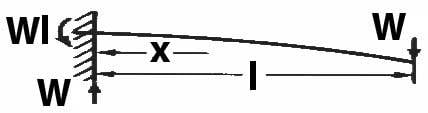I don't understand all this stuff enough to know what to do with the information. What else do I need to find in order to answer the above question?

I have diagrams of the build if anybody is interested in knowing more specifics.

Mentor
Summary:: How much steel needed to support 500 lbs at 40" on a cantilever

Hi, I am trying to figure out how many 1" steel square tubing pieces it would take to support a 500lb weight extended out 40" on a cantilever.

I have seen Modulus of Elasticity figures ranging from .029 GPa to 200 Gpa for Steel. The steel I plan to use would be common hot rolled square tubing. I have not found a figure specific to this material.

I figure my Moment of Inertia to be 20,000 ft-lbs

I've used this information:

Mod of El (lbs/in^2) = 29,000,000
Moment I=wl (in^4) = 20,000
Distance l (in) = 40
Distance x (in) = 32
Distance z (in) = .5

Stress 32" (lbs/in^2) = .1000
Stress 40" (lbs/in^2) = .5000
Deflection 32" (in) = .000
Deflection 40" (in) = .00002
Section Modulus (in^3) = 40000.000

View attachment 268112

I don't understand all this stuff enough to know what to do with the information. What else do I need to find in order to answer the above question?

I have diagrams of the build if anybody is interested in knowing more specifics.
What is your definition of the term "moment of inertia?"

Let's see a typical cross section.

reg7066
The formula I'm using for Moment of Inertia is Weight (W) x Length (l) = Moment of Inertia (M).

M = W x l

I wanted to find a website to augment my answer, but it turns out there are infinite variations on the formula.

(Although this one is not too bad: https://mechanicalc.com/reference/beam-analysis)

I originally got my simple formula from a couple youtube videos, but I'm not sure now which ones.

This seemed pretty cut and dried to me from watching those videos and, originally, your question suprised me, but after combing through the plethora of variations on the net, I can absolutely see why you would ask the question.

I'm not sure how to show you a cross section. The material I would like to use is a 1"x1" hollow square tube. The walls are probably 1/8" thick. This is the common stock at any local hardware store.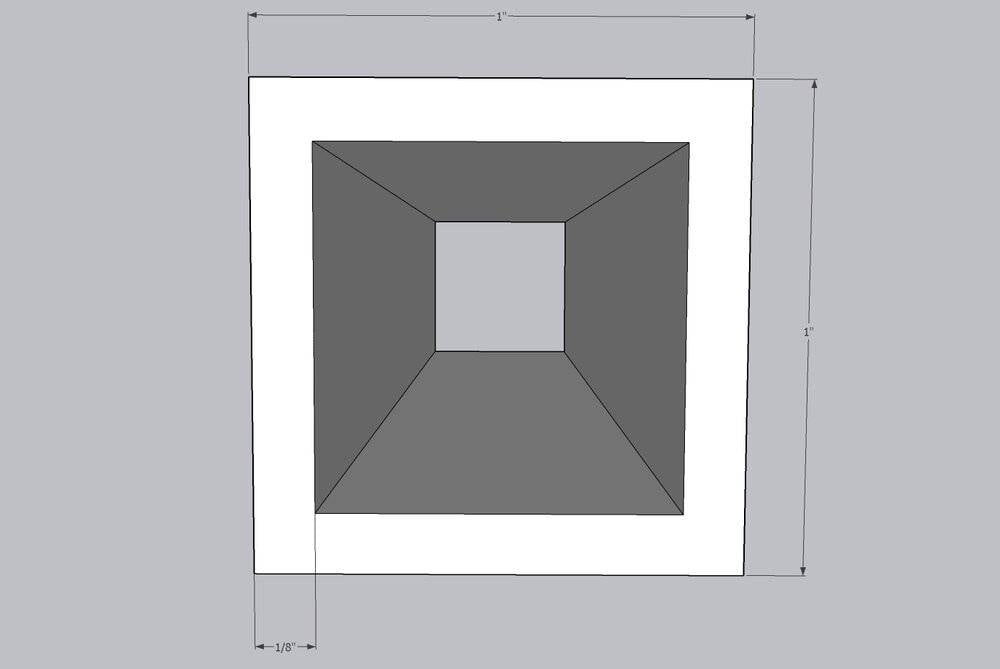It may only be 1/16" thick.

Mentor
The formula I'm using for Moment of Inertia is Weight (W) x Length (l) = Moment of Inertia (M).

M = W x l

I wanted to find a website to augment my answer, but it turns out there are infinite variations on the formula.

(Although this one is not too bad: https://mechanicalc.com/reference/beam-analysis)

I originally got my simple formula from a couple youtube videos, but I'm not sure now which ones.

This seemed pretty cut and dried to me from watching those videos and, originally, your question suprised me, but after combing through the plethora of variations on the net, I can absolutely see why you would ask the question.

I'm not sure how to show you a cross section. The material I would like to use is a 1"x1" hollow square tube. The walls are probably 1/8" thick. This is the common stock at any local hardware store.

View attachment 268114

It may only be 1/16" thick.
That is not the definition of the moment of inertia of the cross section I. That is the moment of the weight force with respect to x = 0. The moment of inertia of a beam of square cross section is ##I=\frac{s^4}{12}##. So the moment of inertia of a beam of hollow cross section is ##I=\frac{s_o^4-s_i^4}{12}##, where the subscripts refer to the outside and inside surfaces.

At a distance x from the built-in end of the beam, the bending moment M(x) is M=W(L-x), so that, at x = 0, M = WL and at x = L, M = 0. The displacement equation for the beam is $$EI\frac{d^2y}{dx^2}=M$$where y is the downward displacement. For a cantilever beam, this is subject to the initial conditions y(0) = 0, y'(0)=0. What do you get for y(x) which you integrate the differential equation subject to these initial conditions?

Mentor
In order to solve this problem, you need to find or calculate the following:

1) The moment of inertia and/or the section modulus.

2) The bending moment.

3) The yield stress.

4) The bending stress.

5) The modulus of elasticity.

6) The deflection.

In all cases, show the equations and the units.

Hint: Do it in the above order. Show your calculations for each step. With correct units.
Hint: Spend some time learning what each of the above terms mean.
Hint: All steel has the same modulus of elasticity.
Hint: You need the exact dimensions to get the moment of inertia and section modulus. Exact wall thickness is critical. Measurement with a ruler is not good enough.
Hint: Use consistent units for all diagrams and calculations. Inches and pounds are good. Mixing feet and inches leads to mistakes.
Hint: There is exactly one equation for the moment of inertia, the section modulus, the bending moment, the bending stress, and the deflection. Various sources will give different values for the yield stress, but they will all be similar.
Hint: Your numbers for the section modulus, stress, and deflection are wrong by several orders of magnitude.

What is the application? Show us a sketch of the entire system so we can check your diagram. Is there a safety issue if it fails?

•Lnewqban
reg7066
jrmichler, I have tried to follow your instructions as best as I could.

These are the specs of the tubing I am considering using (as provided by the supplier):
Steel Square Tube A500/A513 (Welded) 1.000 X 1.000 X 0.120

If I found info on a website, I included the url below the statement.

For the first item I used the equation kindly provided by Chestermiller.
I wasn't sure what "s" stood for, so I assumed it was surface area.
I probably messed this up since I didn't really understand it:

Moment of Inertia (I)= Surface Area (s^4 - s^4) / 12
I = (1 - 0.5776^4) / 12 = 0.1113 / 12 = 9.275 x 10^-3 (in^4)

Section Modulus = width x height squared / 6
(S) = (BH^2/6) - (bh^3/6H)
1/6 - ((.76)(.76)^3)/6(1) = 1/6 - .1927/6 = .1667 - .03212 = .01346 in^3
https://en.wikipedia.org/wiki/Section_modulus

Bending Moment (M) = Weight (W) x Length (L)
M = 500lbs x 40" = 20,000 lb-in

Yield Stress = Yield Strength
Steel, API 5L X65 = 448 MPa
Steel, high strength alloy ASTM A514 = 690 MPa
https://en.wikipedia.org/wiki/Yield_(engineering)

Bending Stress = (Bending Moment)(Vertical Distance from Neutral Axis)/Moment of Inertia
(20,000 lb-in)(.5 in)/.009275 ? = (10,000 lb-in^2)/.009275 = 1.078 x 10^3 ?
https://www.thevitruviusproject.com/resources/normal-stress-bending-stress-shear-stress/

Modulus of Elasticity = 200 GPa
https://www.engineeringtoolbox.com/young-modulus-d_417.html

Deflection = 39.64 in
For this I used a website calculator: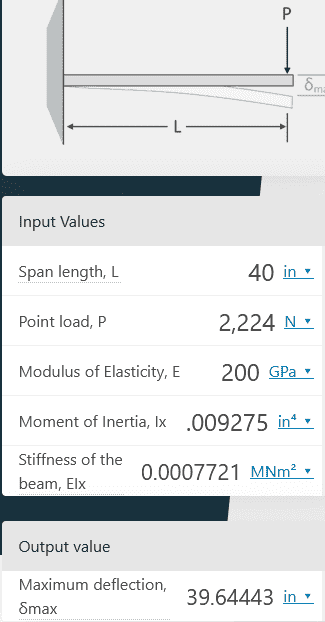https://www.omnicalculator.com/construction/beam-deflection

Chestermiller, the displacement equation is beyond my mental faculties. I haven't done calculus in about 37 years. I'm guessing displacement and deflection are the same thing.

Now for some pics: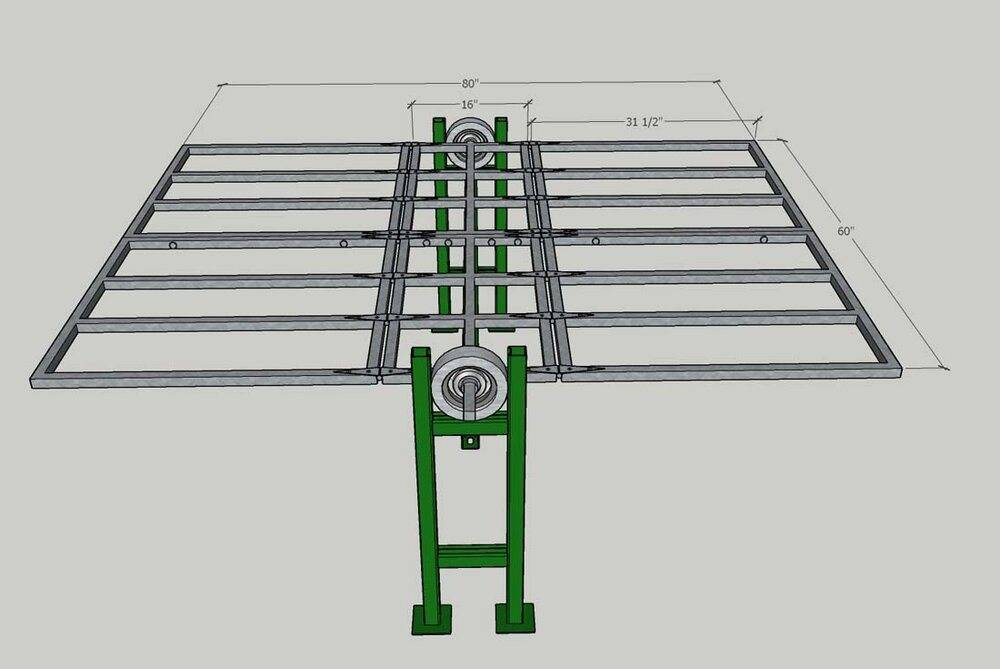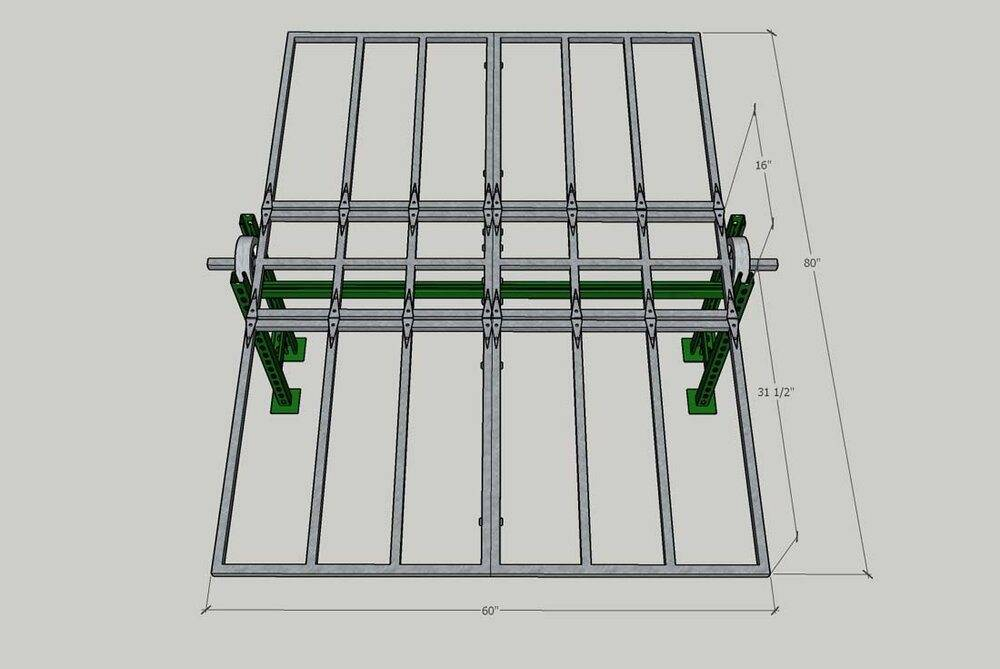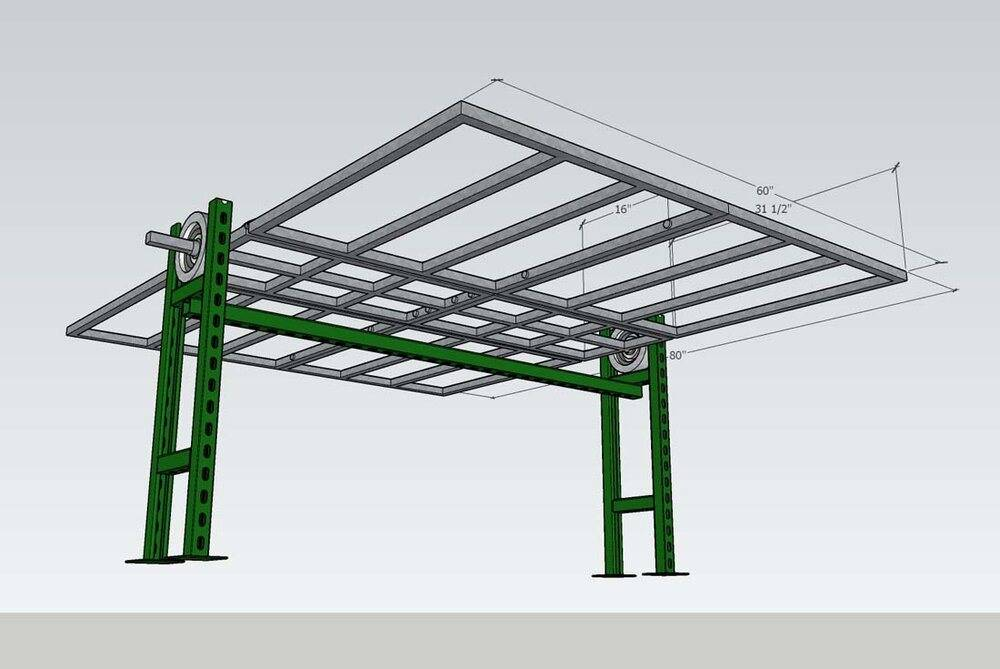What I'm actually trying to build is a work table that will have some flexible options. It will be able to pivot in the center to move a few degrees off of level and it will need to be able to be put into a stowed position when not in use (or semi stowed when only partially in use).

All this information complicates the whole project and initially I just wanted to start with getting the basic info for the size and type of support material. If the numbers for the steel tubing (or other material) are too crazy then there isn't any point in figuring the rest of this out.

The wheels for pivoting and the support stand are only there to help give an idea of the overall intent of the design. I realize that the stand may not be adequate, but there was no point in concerning myself with that before I knew what I was supporting. Likewise the wheels are just what I could easily make using sketchup and not intended to represent a real item or the final product.

Mentor
S is the side of the square.

Mentor
jrmichler, I have tried to follow your instructions as best as I could.

These are the specs of the tubing I am considering using (as provided by the supplier):
Steel Square Tube A500/A513 (Welded) 1.000 X 1.000 X 0.120

If I found info on a website, I included the url below the statement.

For the first item I used the equation kindly provided by Chestermiller.
I wasn't sure what "s" stood for, so I assumed it was surface area.
I probably messed this up since I didn't really understand it:

Moment of Inertia (I)= Surface Area (s^4 - s^4) / 12
I = (1 - 0.5776^4) / 12 = 0.1113 / 12 = 9.275 x 10^-3 (in^4)

Section Modulus = width x height squared / 6
(S) = (BH^2/6) - (bh^3/6H)
1/6 - ((.76)(.76)^3)/6(1) = 1/6 - .1927/6 = .1667 - .03212 = .01346 in^3
https://en.wikipedia.org/wiki/Section_modulus

Bending Moment (M) = Weight (W) x Length (L)
M = 500lbs x 40" = 20,000 lb-in

Yield Stress = Yield Strength
Steel, API 5L X65 = 448 MPa
Steel, high strength alloy ASTM A514 = 690 MPa
https://en.wikipedia.org/wiki/Yield_(engineering)

Bending Stress = (Bending Moment)(Vertical Distance from Neutral Axis)/Moment of Inertia
(20,000 lb-in)(.5 in)/.009275 ? = (10,000 lb-in^2)/.009275 = 1.078 x 10^3 ?
https://www.thevitruviusproject.com/resources/normal-stress-bending-stress-shear-stress/

Modulus of Elasticity = 200 GPa
https://www.engineeringtoolbox.com/young-modulus-d_417.html

Deflection = 39.64 in
For this I used a website calculator:
View attachment 268206
https://www.omnicalculator.com/construction/beam-deflection

Chestermiller, the displacement equation is beyond my mental faculties. I haven't done calculus in about 37 years. I'm guessing displacement and deflection are the same thing.

Now for some pics:

View attachment 268208

View attachment 268209

View attachment 268210

What I'm actually trying to build is a work table that will have some flexible options. It will be able to pivot in the center to move a few degrees off of level and it will need to be able to be put into a stowed position when not in use (or semi stowed when only partially in use).

All this information complicates the whole project and initially I just wanted to start with getting the basic info for the size and type of support material. If the numbers for the steel tubing (or other material) are too crazy then there isn't any point in figuring the rest of this out.

The wheels for pivoting and the support stand are only there to help give an idea of the overall intent of the design. I realize that the stand may not be adequate, but there was no point in concerning myself with that before I knew what I was supporting. Likewise the wheels are just what I could easily make using sketchup and not intended to represent a real item or the final product.
The deflection can't be 39 inches.

The integrated result of the bending equation I presented is:

$$\delta =\frac{WL^3}{3EI}$$where ##\delta## is the displacement at the loaded end of the beam. For a thin walled square like the one in this problem, the moment of inertia equation I presented reduces to $$I=\frac{s^3 t}{3}$$where s is the side of the square and t is the wall thickness. For a 1" square and an 1/8" wall thickness, this gives $$I=\frac{1}{24}\ in^4$$For steel, the elastic modulus in English units is 30000000 psi. What does this give you for ##\delta##?

Last edited:
reg7066
I redid the calculations after correcting the Moment of Inertia from surface area to side of square

Moment of Inertia (I)= Side of Square (s^4 - s^4) / 12

I = (1 - .76^4) / 12 = .6664 / 12 = .05553 in^4

Section Modulus = width x height squared / 6

(S) = (BH^2/6) - (bh^3/6H)

1/6 - ((.76)(.76)^3)/6(1) = 1/6 - .1927/6 = .1667 - .03212 = .01346 in^3

https://en.wikipedia.org/wiki/Section_modulus

Bending Moment (M) = Weight (W) x Length (L)

M = 500lbs x 40" = 20,000 lb-in

Yield Stress = Yield Strength

Steel, API 5L X65 = 448 MPa

Steel, high strength alloy ASTM A514 = 690 MPa

https://en.wikipedia.org/wiki/Yield_(engineering)

Bending Stress = (Bending Moment)(Vertical Distance from Neutral Axis)/Moment of Inertia

(20,000 lb-in)(.5 in)/.05553 in^4 = (10,000 lb-in^2)/.05553 in^4 = 1.800 x 10^5 lb/in^2

https://www.thevitruviusproject.com/resources/normal-stress-bending-stress-shear-stress/

Modulus of Elasticity = 200 GPa

https://www.engineeringtoolbox.com/young-modulus-d_417.html

Deflection = 6.622 in

https://www.omnicalculator.com/construction/beam-deflection

The deflection above looks a lot more reasonable.

Then I tried Chestermillers equation after he reduced it to something I could handle.

I also used .120 for the wall thickness since that was what the supplier provided.

First I converted GPa to Newtons/in^2:

1 N/meter^2 = 0.00064516 N/in^2,

200 GPa = 200,000,000,000 N/m^2 = 129,032,000 N/in^2 = 1.29x10^8 N/in^2

Bending Equation

δ = WL^3/3EI

δ = (2224N)(40in)^3/3(1.29x10^8N/in^2)(.04in^4) = 1.423x10^8N-in^3/1.55x10^7N-in^2 = 9.18 in

This provided slightly different results than the other equation, but they are not that far apart and both are more reasonable than the original mess that I came up with.

6.6 in and 9.2 in

If I'm understanding this correctly this is the deflection I should expect using one stick of tubing and 500 lbs at 40"

10 sticks would get me less than 1" deflection.

Does this make sense?

Mentor
In my judgment, even 1" deflection would be excessive. Have you checked the maximum tensile stress in the beam to see how it compares with the yield stress of steel? I think that you are going to want to have a much higher moment of inertia for the cross section. The 1" hollow cross section seems too flimsy.

reg7066
One of the problems I keep running into is the large range of data for every category.
Here is some tensile strength/yield stress stats that says it is for A500 Steel:

Shaped Structural Tubing

Tensile strength
mn, psi---45 000-----58 000-----62 000-----58 000
(MPa)-----(310)-------(400)-------(427)-------(400)

Yield strength
mn, psi---39 000-----46 000-----50 000-----36 000
(MPa)-----(269)-------(317)-------(345)-------(250)

https://www.tottentubes.com/astm-a500-specification-information

Is this the kind of data you are asking for?

I'm more concerned about failure than deflection. Even though I'm using the figure of 500 lbs, it is unlikely that I'll have anything that heavy. Also, nothing will remain on the extreme end of the table for very long. Once set there, it would immediately be moved to a more central location. I really only care that the table doesn't bend or break.

With that said, I could go with a larger tubing size. 1 1/4" or even 1 1/2" should not change my dimensions too much. I don't have any specs on that at the moment, I will have to contact the supplier again.

reg7066
I found a typo in one of my earlier equations
Bending Equation

δ = WL^3/3EI

δ = (2224N)(40in)^3/3(1.29x10^8N/in^2)(.04in^4) = 1.423x10^8N-in^3/1.55x10^7N-in^2 = 9.18 in

It should be:
δ = (2224N)(40in)^3/3(1.29x10^8N/in^2)(.0555in^4) = 1.42x10^8N-in^3/2.15x10^7N-in^2 = 6.60 in

Which makes both methods of calculating the deflection the same.

I have also done the math for using the next larger size (1 1/4")
Both calculations come out to a deflection of 3.14" for one stick
Presumably this would be .39" if I used the 8 sticks that I show in my drawing.

I'm happy with that for deflection, but I still don't know how to figure out how this relates to the failure point.

Mentor
The maximum tensile strain in any of the cross sections is sy''/2 and the maximum tensile stress is $$Esy''/2=\frac{sM}{2I}$$The maximum bending moment is at the built-in end, and is equal to WL. So the maximum tensile stress is $$\sigma=\frac{WLs}{2I}=\frac{(500)(40)(1)}{(2)(0.05)}=200ksi$$This compares with a tensile strength of 61 ksi. Of course, with 10 bars, that would be reduced to 20 ksi, so it wouldn't exceed the tensile strength. Still, I would rather see a larger safety factor.

reg7066
Chestermiller, thank you for this information.

I might have thought that a factor of 3 would be enough for a safety margin, but I am a novice and I will defer to your judgement.

I took your calculation and plugged in the numbers for 1 1/4" tubing:
(Using the new figure for "I". Moment of Inertia (I)= Side of Square (s^4 - s^4) / 12
I = (1.25^4 - 1.01^4)/12=(2.44 - 1.04)/12 = .117 in^4)

(500)(40)(1.25) 25000
________________ = ________ = 107ksi
(2)(.117) .234

It looks like that alters the maximum tensile stress by a factor of 2.

Did I do this correctly?

If I use 8 bars, that brings it to 13 ksi versus the tensile strength of 61 ksi that you mentioned.
That equals a factor of 4.69.
If this was your table, how much safety margin would you like to have?

Gold Member
What happens when a heavy object rests on one corner of the table? Doesn't that change the element loading, both table and legs?

Cheers,
Tom

reg7066
That is a great observation Tom.G. The only thing I can say is that this worktable is not available to the public. Now that you have pointed that out, if this thing gets built, I'll plan on taping off the corners to prevent that from happening. I really don't need the extreme corners. I could design it with the corners clipped off. Also, if I did have a 500 lb piece to put on the table, it would span roughly 6 square feet. Such a piece would never have all of it's weight on the very edge. Thanks for your input.

Mentor
Chestermiller, thank you for this information.

I might have thought that a factor of 3 would be enough for a safety margin, but I am a novice and I will defer to your judgement.

I took your calculation and plugged in the numbers for 1 1/4" tubing:
(Using the new figure for "I". Moment of Inertia (I)= Side of Square (s^4 - s^4) / 12
I = (1.25^4 - 1.01^4)/12=(2.44 - 1.04)/12 = .117 in^4)

(500)(40)(1.25) 25000
________________ = ________ = 107ksi
(2)(.117) .234

It looks like that alters the maximum tensile stress by a factor of 2.

Did I do this correctly?

If I use 8 bars, that brings it to 13 ksi versus the tensile strength of 61 ksi that you mentioned.
That equals a factor of 4.69.
If this was your table, how much safety margin would you like to have?
It looks like your calculation is correct. But, please exercise safety precautions. I suggest doing several laboratory and prototype tests to make sure that everything is OK. Maybe something like 1 bar using a weight of 25 lbs. You just want to gradually and safely confirm the design.

reg7066
I have taken the structural part of this build as far as I can from a theoretical point of view. Next I need to do some testing. Chestermiller, I will follow your advice and start small. Thank you very much for the enormous amount of help you provided. I couldn't have done it without you.

I know it's off topic, but if anyone wanted to comment or make suggestions on either of my two other quandaries I would welcome it.

1) For the original design to work correctly I need some pretty reliable hinges. I'm pretty sure I can't get anything like that from the local hardware store, so if anyone knows anything about heavy duty hinges, I would love to hear about it. I did play around with a "no-hinge" version that has separate sections that interlace, but that massively increases the complexity of the build and would probably kill me just in welding fees.

2) Another thing I need is a way to hold and release the pivot point. A friend suggested using a break system for a small vehicle. So far that is the best I have.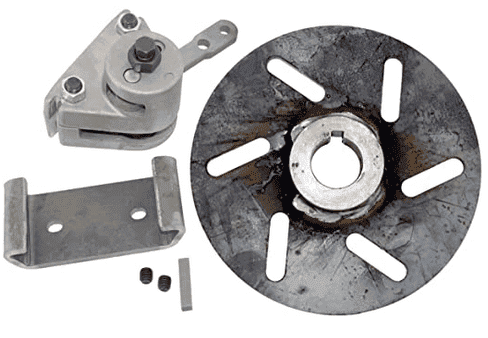Go-Kart Disc Brake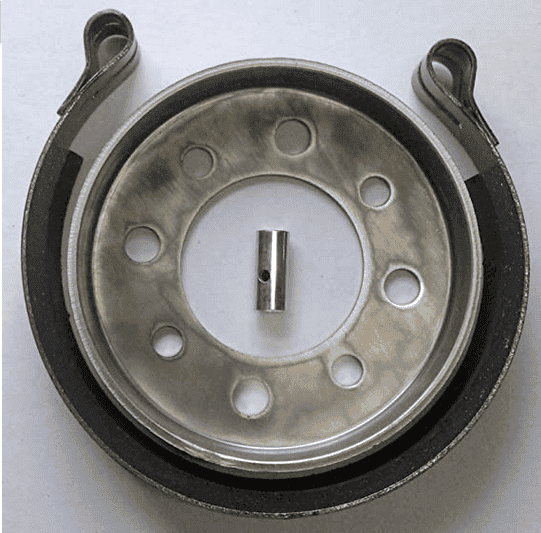Go-Kart Drum Brake

If anybody has any better or just different ideas, I would love to hear them.